Next: Cowling Anti-Dynamo Theorem Up: Magnetohydrodynamic Fluids Previous: Homopolar Generators

# Slow and Fast Dynamos

Let us search for solutions of the MHD kinematic dynamo equation,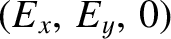(793)

for a prescribed steady-state velocity field,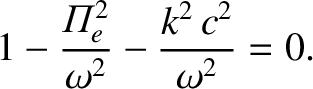, subject to certain practical constraints. Firstly, we require a self-contained solution: i.e., a solution in which the magnetic field is maintained by the motion of the MHD fluid, rather than by currents at infinity. This suggests that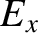as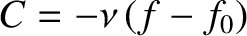. Secondly, we require an exponentially growing solution: i.e., a solution for which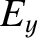, where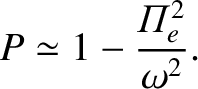.

In most MHD fluids occurring in astrophysics, the resistivity,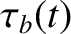, is extremely small. Let us consider the perfectly conducting limit,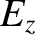. In this limit, Vainshtein and Zel'dovich, in 1978, introduced an important distinction between two fundamentally different classes of dynamo solutions.Suppose that we solve the eigenvalue equation (793) to obtain the growth-rate,, of the magnetic field in the limit. We expect that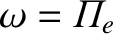(794)

where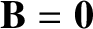. There are two possibilities. Either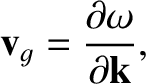, in which case the growth-rate depends on the resistivity, or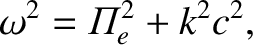, in which case the growth-rate is independent of the resistivity. The former case is termed a slow dynamo, whereas the latter case is termed a fast dynamo. By definition, slow dynamos are unable to operate in the perfectly conducting limit, since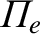as. On the other hand, fast dynamos can, in principle, operate when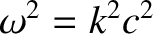.

It is clear, from the above discussion, that a homopolar disk generator is an example of a slow dynamo. In fact, it is easily seen that any dynamo which depends on the motion of a rigid conductor for its operation is bound to be a slow dynamo: in the perfectly conducting limit, the magnetic flux linking the conductor could never change, so there would be no magnetic induction. So, why do we believe that fast dynamo action is even a possibility for an MHD fluid? The answer is, of course, that an MHD fluid is a non-rigid body, and, thus, its motion possesses degrees of freedom not accessible to rigid conductors.

We know that in the perfectly conducting limit () magnetic field-lines are frozen into an MHD fluid. If the motion is incompressible (i.e.,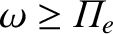) then the stretching of field-lines implies a proportionate intensification of the field-strength. The simplest heuristic fast dynamo, first described by Vainshtein and Zel'dovich, is based on this effect. As illustrated in Fig. 23, a magnetic flux-tube can be doubled in intensity by taking it around a stretch-twist-fold cycle. The doubling time for this process clearly does not depend on the resistivity: in this sense, the dynamo is a fast dynamo. However, under repeated application of this cycle the magnetic field develops increasingly fine-scale structure. In fact, in the limitboth the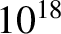andfields eventually become chaotic and non-differentiable. A little resistivity is always required to smooth out the fields on small length-scales: even in this case the fields remain chaotic.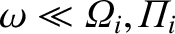At present, the physical existence of fast dynamos has not been conclusively established, since most of the literature on this subject is based on mathematical paradigms rather than actual solutions of the dynamo equation. It should be noted, however, that the need for fast dynamo solutions is fairly acute, especially in stellar dynamo theory. For instance, consider the Sun. The ohmic decay time for the Sun is about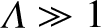years, whereas the reversal time for the solar magnetic field is only 11 years. It is obviously a little difficult to believe that resistivity is playing any significant role in the solar dynamo.

In the following, we shall restrict our analysis to slow dynamos, which undoubtably exist in nature, and which are characterized by non-chaoticandfields.Next: Cowling Anti-Dynamo Theorem Up: Magnetohydrodynamic Fluids Previous: Homopolar Generators
Richard Fitzpatrick 2011-03-31